by Chris Byrd

# Summary

Hypertexture is a method developed by Ken Perlin, a professor at NYU, to model and generate complex 3d textures. It can be a difficult task to model certain types of textures. Expecially those that have incredably complex boundaries like hair or fur, or those that have no real 'fixed' boundaries such as flames or smoke. Hypertexture introduces a fairly simple way of createing these objects. This presentation will give an introduction to how hypertexture works.

# Overview of Presentation

The work Perlin did in procedural texture generation  formed the basis for the work he did in . I will first give an overview of some of the concepts for procedural texture generation. Then I will talk about hypertexture itself, and lastly I will briefly intruduce the ray casting methods used to render hypertexture.

# What is Procedural Texture Mapping?

Procedural texture mapping and procedural modeling is the use of a function or set of functions applied to a set of points in order to generate a texture. One of the most well known methods of procedural texture mapping is probally the use of fractal techniques to generate terrain. There are two main reasons for wanting to use this method to generate textures. One is that because of the use of function to define the texture it is easy to introduce time-varying variable to your model thus creating animation. The other benefit is to be able to specify a highly complex texture with minimal amounts of data storage.

# 3d Textures

There are a couple additional benefits of a procedural approach to generating textures when you move into three dimensions. One of the other possibilities for getting texture onto a three dimensional object is to map a 2D texture onto the 3D object surface. This might work well with simple objects, however once you get 3D objects that exhibit any great amount of complexity useing the 2D mapping technique can become problematic. With procedural mapping all you need to know is a points location in space. Using that location you can determine what the value of the texture field at that point. This method has been called 'solid textureing'. The only limitation to this method is that you need to be able to define the texture by a set of functions. So mapping a picture taken from a digital camera would not be possible using this method.

One of the common solid texturing techniques is to use a noise function to simulate turbulence. Perlin developed some turbulence models for solid texturing and he extended them to also work with hypertexture. A more in depth talk about noise generation was given by Prof. Ward here . The basic idea is to define a 3D array(lattice) of random numbers. The amount of noise applied to any spot is the random value located at the corresponding location in the array. If the location in space does not correspond with an exact location in the array, various different interpolation methods can be applied to approximate the value using neighboring values in the lattice. Turbulence is defined as summation of noise useing the following equation:
Using this model many realistic textures, such as those of marble or flames can be created.

# Hypertexture

Hypertexture is an extension of the solid textures. One of the main distinctions between sold textures and hypertexture is that hypertexture objects have no well defined boundaries. Instead thay have a density function that describes how the object should behave in the area where it transitions between the outside and inside of the object.

An object in Hypertexture is partitioned into three different regions. A hard region, a soft region and an outside region. The hard region represents a part of the object where it is completely solid. The soft region represents a part of the object where the density is variable, and the outside region represents where the object is non-existant. The essence of hypertexture is the definition of functions that modify the behavior of the density of an object within its soft region.

There are two main functions that define a hypertexture. Those are the Object Density Function, D(x), and the Density Modulation Function (DMF), f.

The Density function, D(x), describes the density of the object for all points thoughout R^3. D(x) has a range of of 0 to 1. A value of 1 means the object is completely solid at that point. A value of 0 means the object has no density at that point. All values of D(x) such that 0 < D(x) < 1, represent the objects soft region.

The Density Modulation Function are the functions that control the behavior of the density within an objects soft region. Perlin defines a set of base DMF's that can be used as building blocks to define any hypertexture you want, the functions he defines will be discussed shortly. A DMF is applied to an objects density function. Multiple DMF's can be applied to the same D(x).

Hypertexture is formally defined as:

H(D(x),x)= fn(...f2(f1(f0(D(x))))) Where f0, f1, ... fn are all DMF's.

# An example D(x)

The following is an example of a D(x) for a sphere. Given D[c,r,s](x), where c is the center of a sphere, r is is radios and s is the width of its soft region.
```D(x) =
r1 = inner_radius^2 = (r - s/2)^2
r2 = outer_radius^2 = (r + s/2)^2
rx = distance_from_center = (x.x - c.x)^2 + (x.y - c.y)^2 + (x.z - c.z)^2
if(rx<=r1) then 1.0
else if(rx>=r2) then 0.0
else (r1-rx)/(r2-r1) /* replace this with whatever you want the density to look like*/

```

In order to be able to add together various objects soft objects the following boolean functions were defined.

The intersection of two density funcions D1(x) and D2(x) is D1(x)D2(x).

The complement of a density funcions D(x) is 1-D(x).

The difference between two density funcions D1(x) and D2(x) is D1(x) - D1(x)D2(x).

The union of two density funcions D1(x) and D2(x) is D1(x) + D2(x) - D1(x)D2(x).

# Density Modulation Functions

Density modulation functions are used to shape the behavior of an objects density inside of its soft region. The DMF that will be described here are the bias, gain, noise and turbulence functions

## Bias functions

The bias function is used to bend the Density function either upwards or downwards over the [0,1] interval. The rules the bias function has to follow are:

bias(b,0)=0

bias(b,.5)=b

bias(b,1)=1

The following function exhibits those properties:

bias(b,t) = t^(ln(b)/ln(0.5))

You can see a the bias curve of a couple different values here: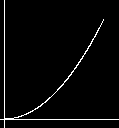A Bias of 0.25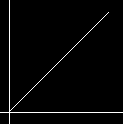A Bias of 0.7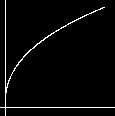A Bias of 0.75

## Gain functions

The gain function is used to help shape how fast the midrange of an objects soft region goes from 0 to 1. A higher gain value means the a higher rate in change. The rules of the gain function are as follows:

gain(g,0)=0

gain(g,1/4)=(1-g)/2

gain(g,1/2)=1/2

gain(g,3/4)=(1+g)/2

gain(g,1)=1

The gain function is defined as a spline of two bias curves: gain(g,t)= if (t<0.5) then bias(1-g,2*t) else 1-bias(1-g,2-2*2t)/2

You can see a the bias curve of a couple different values here: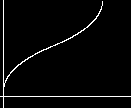A gain of 0.25A gain of 0.7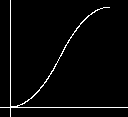A gain of 0.75

Just so you can visualize the effect of combining these functions a bit better the following graphs show the effect of both gain and bioa on a line. The following two graphs show the sort of change in density you could expect by combining gain and bias functions.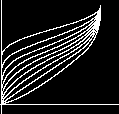f1(x)= gain(.25,bias(j,i))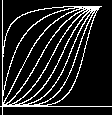f2(x)= gain(.75,bias(j,i)

The two functions f1(x) and f2(x) are graphed with varying degree of bias. f1(x) has a high gain value, f2(x) has a low gain value. In both functions that bias is brought from .1 to .9. As you can see in the graphs a change in gain affects how fast the graph changes density, while the changes in bias affect how high or low the overall density there is.

# Noise

Noise generation for hypertexture is somewhat similar to how noise generation was done with solid textueing. Noise is implemented by indexing into a 3D array of pseudorandom vectors uniformly distributed on the units sphere, finding the surrounding 8 integer points and performing interpolation on those points to find your value. This method will return a value between -1 and 1.

Perlin implements his indexing function in the folowing way:

G is the lattice of pseudorandom unit vectors.

He creates an array P consisting of a random permutation of the first n integers. (where n is the size of the G array, typically 256).

The gradient is defined as follows:

T[i,j,k] = G( O(i + O(j + O(k))));

Where O(i) is defined as:

O(i) = P[i % n ]

This [i,j,k]'s 8 neighbors are then found and hermite interpolation is performed on them.

This interpolation is accomplished by taking the dot product of the gradient and the location in the lattice (i,j,h) and evaluating that value using the hermite derivative basis function:

f(x)= 3t^2 - 2t^2

This value is then used to interpolate between each first x then y and finally z.

Given g1 and g2 (two gradients dotted with their lattice location), you interpolate using the formula: Interpolate = g1 + hermite * (g1 - g2) )

The unit vectors in G are computed useing the following algorithm:
```(1) Generate a random vector within the interval [-1,1]
(2) if |v| > 1 then goto 1
(3) Normalize the length of v
```

# Turbulence

Turbulence is defined same was as it was in soldid texturing, a summation of noise:# Rendering Hypertextures

Hypertexture is rendered using volume rendering techniques. This is the easiest method because hypertexture objects do not always have well defined surfaces.

A ray marching algorithm is used to render hypertexture images. A ray is cast into each pixel in space, on each ray it is tested wether or not that ray intersects the parallelpiped that bounds the hypertexture object. If the ray does intersect with the bounding volume then its entry and exit points are calculated. We begin at the entry point and and 'march' down the ray in some increment, sampling values along the way. We keep doing this till we hit the exit point of the parallelpiped. The points along the ray that we stop along are defined as follows:

x = x_0 + K * delta_u

Along each sampleing point of the ray, we calculate the DMF, f(x) at that location. If 0 < f(x) < 1 then the gradient at that point is estimated and then used to calculate the normal vector for that point. The normal vector can in turn be used to calculate things like shading, reflection etc.

To compute the gradient we need 3 points that are perpendicular to each other. The first point can be f(x-delta_u) since that is already computed. The other two vectors delta_v and delta_w are any other two vectors that are all mutually perpendicular. We put these together to give us the vector:

gradient_f = [ f(x) - f(x-delta_u), f(x+delta_v)-f(x), f(x+delta_w)+f(x) ]

This resulting vector is in (u,v,w) space, to convert it to (x,y,z) space it is multiplied by the following matrix:

```| delta_x  |
| delta_v  |
| delta_w  |
```

The other thing that needs to be done along the ray march is to collect information about opacity. This is done by summing up the color and opacity of the sampleing point as we march down the ray using the following formula:

```	t = o_k (1-o)
color = color + t*color_k
o_k = o + t
```

Where o_k and color_k are the opacity and collor of the kth step down the ray.

One of the benefits over a front to back approach to raymarching is that once opacity reaches 100% we can stop calculating it. Rendering Hypertexture is a very time consuming task, it is O(n^3). Where n is the resolution of the image. Performace withing the soft region of an object is 3 times as expensive as that of outide the soft region because we are calculating f(x) 3 times on each step.

# Images

:

Some images I made useing the DMF's given: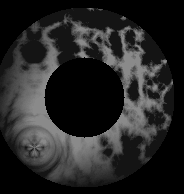Turbulent sphere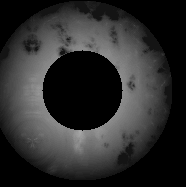Same sphere, different bias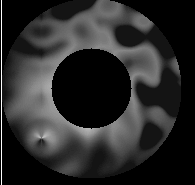Noisy sphere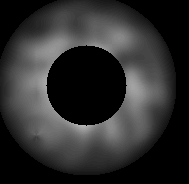Another Noisy sphere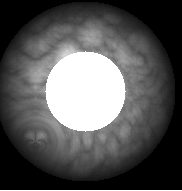Some hypertexture images

References:
``` Perlin, K Hypertexture, Computer Graphics 1989, 253-62
 Perlin, K. An Image Synthesizer. Computer Graphics 1985, 287-296
http://www.mrl.nyu.edu/hyper/hypertexture/hypertexture.ps
 Alan Watt, Mark Watt. Advanced Animation and Rendering Techniques
```